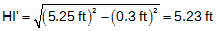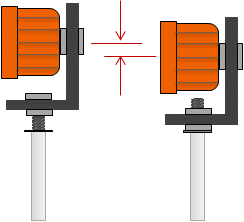### 3. Errors

Basically, the errors described in the Errors chapter of the Electronic Distance Measurement topic also affect trigonometric leveling. In addition, those errors arising from TSI zenith angle measurements will have an effect on elevation determination. Those aspects are a function of the angle measurement capacity of the TSI and will be described in the Errors chapter of the TSI topic.

Other errors by source -

##### (1) Personal

Most personal errors are generally in:

• determining HI or HR
• recording
• computations
##### (2) Instrumental

(a) Taping HI

A shown in Figure F-4, measuring the HI with a tape introduces an error since the tape can't be used to measure vertically. How much error? Assuming an approximate 8 inch TSI width and a tape distance of 5.25 ft, the true HI can be computed from geometry shown in Figure F-7.In this case, an error of only 0.02 ft is introduced in the TSI elevation. That's not much although not up to diffential leveling. Figure F-7 HI Measurement Error

Because a prism pole is used extensively in trigonometric leveling we need to understand some potential errors associated with it and how they can be compensated.

If the prism pole is set to 5.70 ft, is it really 5.70 ft from the bottom of the rod to the center of the reflector, Figure F-8?Figure F-8 Prism Pole Uncertainty

There are a few reasons the prism pole reading may be incorrect:

 How the reflector is mounted. Most screw onto the top of the pole and are held in place using a lock nut. Depending on how far down the reflector is screwed will affect its height above the ground.Figure F-9 Prism Mounting Height Difference The type of reflector used. Different reflector designs, single versus multiple reflectors, have different center locations.Figure F-10 Prism Type Height Difference Pole tip. A regular pointed tip can sink in soft ground changing the reflector height. A topo shoe, having a blunt tip, is often used instead of the pointed tip. The blunt tip prevents the pole sinking in the ground maintaing a consistent HR regardless the terrain. But... The height of the topo shoe may be different than the pointed tip which could affect the pole height reading accuracy.Figure F-11 Pole Bottom Height Difference

If a tape or pocket-rod is used to measure HI, it should also be used to set the HR.

For example, assume the HI measured with a tape is 5.35 ft, Figure F-12(a). With the reflector set to the same height using the tape, the prism pole reading is 5.52 ft, Figure F-12(b). Had the HR been set using a 5.35 ft prism pole reading, there would be almost 0.2 ft error in each measured elevation. The difference between the HI and the reading on the prism pole is a systematic error.(a) Taped Height (b) Prism Height Figure F-12 Prism Height Systematic Error

If the prism pole is used to measure the HI, then as long as the same pole is used to measure points any height error is compensated. That's because the error is added in the HI and subtracted in the HR to determine point elevations.

However, an HI error can be introduced if the if the ground isn't flat, Figure F-13, making the pole bottom higher or lower than the point.Figure F-13 Uneven Ground Slope

In those situations a tape should be used to determine the HI and to set the reflector height.

If sighting a reference BM to establish the TSI elevation, Figure F-6, then pole reading error is automatically compensated as long as the same pole is used throughout. The pole height error is added to obtain the TSI elevation, then subtracted for point elevations. However, the TSI elevation may be incorrect subject to the pole reading error in the HRBM.

(c) Rod bubble

The prism pole not being truly vertical has a greater effect on horizontal distance than vertical distance measurement. That's not to say a maladjusted bubble should be used for trigonometric leveling because that operation is generally combined with horizontal angles and distances to perform three dimensional surveys. The rod bubble on the prism pole should be checked and adjusted as described in the Errors chapter of Electronic Distance Measurement

##### (3) Natural

The same environmental conditons affecting distance measurement and angle measurement also affect trigonometric leveling.